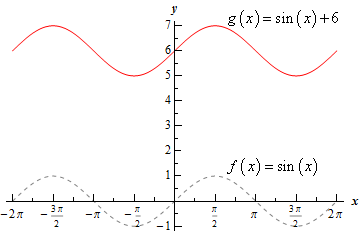Paul's Online Notes
Home / Calculus I / Review / Common Graphs
Show Mobile Notice Show All Notes Hide All Notes
Mobile Notice
You appear to be on a device with a "narrow" screen width (i.e. you are probably on a mobile phone). Due to the nature of the mathematics on this site it is best views in landscape mode. If your device is not in landscape mode many of the equations will run off the side of your device (should be able to scroll to see them) and some of the menu items will be cut off due to the narrow screen width.

### Section 1.10 : Common Graphs

3. Without using a graphing calculator sketch the graph of $$g\left( x \right) = \sin \left( x \right) + 6$$.

Hint : Recall that the graph of $$f\left( x \right) + c$$ is simply the graph of $$f\left( x \right)$$ shifted down by $$c$$ units if $$c < 0$$ or shifted up by $$c$$ units if $$c > 0$$.
Show Solution

Recall the basic Algebraic transformations. If we know the graph of $$f\left( x \right)$$ then the graph of $$f\left( x \right) + c$$ is simply the graph of $$f\left( x \right)$$ shifted down by $$c$$ units if $$c < 0$$ or shifted up by $$c$$ units if $$c > 0$$.

So, in our case if $$f\left( x \right) = \sin \left( x \right)$$ we can see that,

$g\left( x \right) = \sin \left( x \right) + 6 = f\left( x \right) + 6$

and so the graph we’re being asked to sketch is the graph of the sine function shifted up by 6 units.

Here is the graph of $$g\left( x \right) = \sin \left( x \right) + 6$$ and note that to help see the transformation we have also sketched in the graph of $$f\left( x \right) = \sin \left( x \right)$$.